true

Nitin Pathak

Pimple Saudagar, Pune, India - 411027# Nitin Pathak

Pimple Saudagar, Pune, India - 411027.

5 Students taught

Referral Discount: Get ₹ 500 off when you make a payment to start classes. Get started by Booking a Demo.

Details verified of Nitin Pathak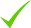IdentityEducation

Know how UrbanPro verifies Tutor details

Identity is verified based on matching the details uploaded by the Tutor with government databases.

## Overview

Nitin Pathak conducts classes in Class 10 Tuition, Class 11 Tuition and Class 12 Tuition. Nitin is located in Pimple Saudagar, Pune. Nitin takes at students Home, Regular Classes- at his Home and Online Classes- via online medium. He has 2 years of teaching experience . Nitin has completed Bachelor of Engineering (B.E.) from Surat in 2014. He is well versed in English, Hindi, English and English.

## Languages Spoken

Hindi

English Proficient

English Proficient

English Proficient

## Education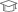Surat 2014

Bachelor of Engineering (B.E.)

Pimple Saudagar, Pune, India - 411027

## Verified Info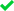ID VerifiedPhone VerifiedEmail VerifiedFacebook Verified

Report this Profile

Is this listing inaccurate or duplicate? Any other problem?

Type the letters as shown below *

Please enter the letters as show below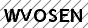Class 11 Tuition

Class LocationOnline (video chat via skype, google hangout etc)Student's HomeTutor's Home

Years of Experience in Class 11 Tuition

2

Board

State, International Baccalaureate, IGCSE, CBSE, ISC/ICSE

IB Subjects taught

Physics, Mathematics, Hindi

ISC/ICSE Subjects taught

Hindi, Mathematics, Physics

CBSE Subjects taught

Physics, Mathematics

IGCSE Subjects taught

Physics, Mathematics, Hindi

Taught in School or College

No

State Syllabus Subjects taught

Mathematics, Physics, Hindi

## Reviews

this is test message this is test message this is test message this is test message this is test message this is test message this is test message

No Reviews yet! Be the first one to Review

## FAQs

1. Which school boards of Class 12 do you teach for?

State, International Baccalaureate, IGCSE and others

2. Have you ever taught in any School or College?

No

3. Which classes do you teach?

I teach Class 10 Tuition, Class 11 Tuition, Class 12 Tuition, Class 6 Tuition, Class 7 Tuition, Class 8 Tuition and Class 9 Tuition Classes.

4. Do you provide a demo class?

Yes, I provide a free demo class.

5. How many years of experience do you have?

I have been teaching for 2 years.

## Answers by Nitin Pathak (15)

Answered on 25/07/2019 CBSE/Class 10/Mathematics/UNIT IV: Geometry/Triangles/NCERT Solutions/Exercise 6.5

Given : AB = 6√3cm AC= 12 cm BC=6 cm Ans : check if (AC)²=(BC)²+(AB)² 12²=(6)²+(6√3)² 144= 36+108 144=144 Angle B is 90º Option (C) is correct
Dislike Bookmark

Answered on 25/07/2019 CBSE/Class 10/Mathematics/UNIT IV: Geometry/Triangles/NCERT Solutions/Exercise 6.5

Two poles of heights 6 m and 11 m stand on a plane ground. If the distance between the feet of the poles... ...more
Two poles of heights 6 m and 11 m stand on a plane ground. If the distance between the feet of the poles is 12 m, find the distance between their tops.

Given : distance btween two poles = 12 m Small pole height = 6 m Big pole height = 11 m To find : distnace btween two poles Small to top pole distance can be find by pythgores therom top (hypotenuse)²=(base)²+(height)² Base =12 m Height = 11-6= 5 m (Hypotenuse)²=(12)²+(5)² Hypotenuse... ...more

Given : distance btween two poles = 12 m

Small pole height = 6 m

Big pole height = 11 m

To find : distnace btween two poles

Small to top pole distance can be find by pythgores therom top (hypotenuse)²=(base)²+(height)²

Base =12 m

Height = 11-6= 5 m

(Hypotenuse)²=(12)²+(5)²

Hypotenuse =√(12)²+(5)²m

Hypotenuse=√144+25= √169m

distance between their tops is13 m.

Dislike Bookmark

Answered on 24/07/2019 CBSE/Class 10/Mathematics/UNIT I: Number systems/Real numbers/NCERT Solutions/Exercise 1.3

1) 1⁄√2 Rationalise this number 1 *√2⁄√2 *√2 = √2/2 √2 is irrational number and 2 is rational number division of rational number by irrational number is irrational . Hence 1/√2 is an irrational number. 2)7√5 7 is rational number √5... ...more

1) 1⁄√2

Rationalise this number 1 *√2⁄√2 *√2

= √2/2

√2 is irrational number and 2 is rational number division of rational number by irrational number is irrational . Hence  1/√2 is an irrational number.

2)7√5

7 is rational number √5 is an irrational number product of rarional and irrational number is irrational number.

3)6+√5

6 is a rational number √5 is irrational number sum of rational and irrational number is an irrational number.

Dislike Bookmark

Answered on 24/07/2019 CBSE/Class 10/Mathematics/UNIT I: Number systems/Real numbers/NCERT Solutions/Exercise 1.3

To prove : 3+2√5 is rational number. Ans: 3 is rational number. 2 is rational number. 2√5 is irrational number (product of rational and irrational is an irrational number) 3+2√5 is an irrational number (sum of rational and irrational number is irrational) Or Let P = 3+2√5 is... ...more

To prove : 3+2√5 is rational number.

Ans: 3 is rational number.

2 is rational number.

2√5 is irrational number (product of rational and irrational is an irrational number)

3+2√5 is an irrational number (sum of rational and irrational number is irrational)

Or

Let P = 3+2√5 is rational number ( we are assuming )

P= 3+2√5

P-3 =2√5

(P-3)÷2=√5

L.H.S. we assume P is a rational number .

P-3 is also rational number substraction of rational number always rational number .

(P-3)÷2 rational number (P-3) divide by rational number 2 hence (P-3)÷2 is rational number .

L.H.S. is rational While R.H.S. √5 is irrational L.H.S ≠R.H.S.

Our assumption is wrong so we can say 3+2√5 is an irrational number.

Dislike Bookmark

Hi, If you are comfortable with online mode then go with online coaching but if good options are available for offline coaching in your area then prefer that.
Dislike Bookmark
Class 11 Tuition 5.0

Class LocationOnline (video chat via skype, google hangout etc)Student's HomeTutor's Home

Years of Experience in Class 11 Tuition

2

Board

State, International Baccalaureate, IGCSE, CBSE, ISC/ICSE

IB Subjects taught

Physics, Mathematics, Hindi

ISC/ICSE Subjects taught

Hindi, Mathematics, Physics

CBSE Subjects taught

Physics, Mathematics

IGCSE Subjects taught

Physics, Mathematics, Hindi

Taught in School or College

No

State Syllabus Subjects taught

Mathematics, Physics, Hindi

Class 9 Tuition 5.0

Class LocationOnline (video chat via skype, google hangout etc)Student's HomeTutor's Home

Years of Experience in Class 9 Tuition

2

Board

CBSE, International Baccalaureate, State, IGCSE, ICSE

IB Subjects taught

Physics, Hindi, Mathematics

CBSE Subjects taught

Mathematics, Hindi, Science

ICSE Subjects taught

Hindi, Physics

IGCSE Subjects taught

Mathematics, Physics

Taught in School or College

No

State Syllabus Subjects taught

Mathematics, Hindi, Science

Class 10 Tuition 5.0

Class LocationOnline (video chat via skype, google hangout etc)Student's HomeTutor's Home

Years of Experience in Class 10 Tuition

2

Board

CBSE, International Baccalaureate, State, IGCSE, ICSE

IB Subjects taught

Physics, Hindi, Mathematics

CBSE Subjects taught

Mathematics, Hindi, Science

ICSE Subjects taught

Hindi, Physics

IGCSE Subjects taught

Mathematics, Physics

Taught in School or College

No

State Syllabus Subjects taught

Mathematics, Hindi, Science

Class 8 Tuition 5.0

Class LocationOnline (video chat via skype, google hangout etc)Student's HomeTutor's Home

Years of Experience in Class 8 Tuition

2

Board

State, International Baccalaureate, IGCSE, ICSE, CBSE

IB Subjects taught

Mathematics, Science, Hindi

CBSE Subjects taught

Science, Mathematics, Hindi

ICSE Subjects taught

Physics, Hindi, Mathematics

IGCSE Subjects taught

Science, Mathematics

Taught in School or College

No

State Syllabus Subjects taught

Science, Mathematics, Hindi

Class 12 Tuition 5.0

Class LocationOnline (video chat via skype, google hangout etc)Student's HomeTutor's Home

Years of Experience in Class 12 Tuition

2

Board

State, International Baccalaureate, IGCSE, CBSE, ISC/ICSE

IB Subjects taught

Physics, Mathematics, Hindi

ISC/ICSE Subjects taught

Hindi, Mathematics, Physics

CBSE Subjects taught

Physics, Mathematics

IGCSE Subjects taught

Physics, Mathematics, Hindi

Taught in School or College

No

State Syllabus Subjects taught

Mathematics, Physics, Hindi

Class 7 Tuition 5.0

Class LocationOnline (video chat via skype, google hangout etc)Student's HomeTutor's Home

Years of Experience in Class 7 Tuition

2

Board

State, International Baccalaureate, IGCSE, ICSE, CBSE

IB Subjects taught

Mathematics, Science, Hindi

CBSE Subjects taught

Science, Mathematics, Hindi

ICSE Subjects taught

Physics, Hindi, Mathematics

IGCSE Subjects taught

Science, Mathematics

Taught in School or College

No

State Syllabus Subjects taught

Science, Mathematics, Hindi

Class 6 Tuition 5.0

Class LocationOnline (video chat via skype, google hangout etc)Student's HomeTutor's Home

Years of Experience in Class 6 Tuition

2

Board

State, International Baccalaureate, IGCSE, ICSE, CBSE

IB Subjects taught

Mathematics, Science, Hindi

CBSE Subjects taught

Science, Mathematics, Hindi

ICSE Subjects taught

Physics, Hindi, Mathematics

IGCSE Subjects taught

Science, Mathematics

Taught in School or College

No

State Syllabus Subjects taught

Science, Mathematics, Hindi

this is test message this is test message this is test message this is test message this is test message this is test message this is test message

No Reviews yet! Be the first one to Review

## Answers by Nitin Pathak (15)

Answered on 25/07/2019 CBSE/Class 10/Mathematics/UNIT IV: Geometry/Triangles/NCERT Solutions/Exercise 6.5

Given : AB = 6√3cm AC= 12 cm BC=6 cm Ans : check if (AC)²=(BC)²+(AB)² 12²=(6)²+(6√3)² 144= 36+108 144=144 Angle B is 90º Option (C) is correct
Dislike Bookmark

Answered on 25/07/2019 CBSE/Class 10/Mathematics/UNIT IV: Geometry/Triangles/NCERT Solutions/Exercise 6.5

Two poles of heights 6 m and 11 m stand on a plane ground. If the distance between the feet of the poles... ...more
Two poles of heights 6 m and 11 m stand on a plane ground. If the distance between the feet of the poles is 12 m, find the distance between their tops.

Given : distance btween two poles = 12 m Small pole height = 6 m Big pole height = 11 m To find : distnace btween two poles Small to top pole distance can be find by pythgores therom top (hypotenuse)²=(base)²+(height)² Base =12 m Height = 11-6= 5 m (Hypotenuse)²=(12)²+(5)² Hypotenuse... ...more

Given : distance btween two poles = 12 m

Small pole height = 6 m

Big pole height = 11 m

To find : distnace btween two poles

Small to top pole distance can be find by pythgores therom top (hypotenuse)²=(base)²+(height)²

Base =12 m

Height = 11-6= 5 m

(Hypotenuse)²=(12)²+(5)²

Hypotenuse =√(12)²+(5)²m

Hypotenuse=√144+25= √169m

distance between their tops is13 m.

Dislike Bookmark

Answered on 24/07/2019 CBSE/Class 10/Mathematics/UNIT I: Number systems/Real numbers/NCERT Solutions/Exercise 1.3

1) 1⁄√2 Rationalise this number 1 *√2⁄√2 *√2 = √2/2 √2 is irrational number and 2 is rational number division of rational number by irrational number is irrational . Hence 1/√2 is an irrational number. 2)7√5 7 is rational number √5... ...more

1) 1⁄√2

Rationalise this number 1 *√2⁄√2 *√2

= √2/2

√2 is irrational number and 2 is rational number division of rational number by irrational number is irrational . Hence  1/√2 is an irrational number.

2)7√5

7 is rational number √5 is an irrational number product of rarional and irrational number is irrational number.

3)6+√5

6 is a rational number √5 is irrational number sum of rational and irrational number is an irrational number.

Dislike Bookmark

Answered on 24/07/2019 CBSE/Class 10/Mathematics/UNIT I: Number systems/Real numbers/NCERT Solutions/Exercise 1.3

To prove : 3+2√5 is rational number. Ans: 3 is rational number. 2 is rational number. 2√5 is irrational number (product of rational and irrational is an irrational number) 3+2√5 is an irrational number (sum of rational and irrational number is irrational) Or Let P = 3+2√5 is... ...more

To prove : 3+2√5 is rational number.

Ans: 3 is rational number.

2 is rational number.

2√5 is irrational number (product of rational and irrational is an irrational number)

3+2√5 is an irrational number (sum of rational and irrational number is irrational)

Or

Let P = 3+2√5 is rational number ( we are assuming )

P= 3+2√5

P-3 =2√5

(P-3)÷2=√5

L.H.S. we assume P is a rational number .

P-3 is also rational number substraction of rational number always rational number .

(P-3)÷2 rational number (P-3) divide by rational number 2 hence (P-3)÷2 is rational number .

L.H.S. is rational While R.H.S. √5 is irrational L.H.S ≠R.H.S.

Our assumption is wrong so we can say 3+2√5 is an irrational number.

Dislike Bookmark

Hi, If you are comfortable with online mode then go with online coaching but if good options are available for offline coaching in your area then prefer that.
Dislike Bookmark

Nitin Pathak conducts classes in Class 10 Tuition, Class 11 Tuition and Class 12 Tuition. Nitin is located in Pimple Saudagar, Pune. Nitin takes at students Home, Regular Classes- at his Home and Online Classes- via online medium. He has 2 years of teaching experience . Nitin has completed Bachelor of Engineering (B.E.) from Surat in 2014. He is well versed in English, Hindi, English and English.

•• Want to learn from Nitin Pathak?

• Contact Now
X

Recommended Profiles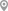Pimple Saudagar, PunePimple Saudagar, PunePimple Saudagar, PunePimple Saudagar, PunePimple Saudagar, PunePimple Saudagar, Pune

X

Let us shortlist and give the best tutors and institutes.

or

Send Enquiry to Nitin Pathak

Let Nitin Pathak know you are interested in their class